# Transformation of Curves, Surfaces and Volume Civil Engineering (CE) Notes | EduRev

## Civil Engineering (CE) : Transformation of Curves, Surfaces and Volume Civil Engineering (CE) Notes | EduRev

The document Transformation of Curves, Surfaces and Volume Civil Engineering (CE) Notes | EduRev is a part of the Civil Engineering (CE) Course Advanced Solid Mechanics - Notes, Videos, MCQs & PPTs.
All you need of Civil Engineering (CE) at this link: Civil Engineering (CE)

Transformation of curves, surfaces and volume
Before rigorously deriving the general expressions for transformation of curves, surfaces and volumes, let us obtain these expressions after various simplifying assumptions.
Let us assume the following:
1. The same Cartesian coordinate basis and origin is used to describe the body in both its reference and current configuration.
2. The body is subjected to displacement u and the Lagrangian description of the displacement field is known and is sufficiently smooth for the required derivatives to exist. That is

u = ux(X, Y, Z)ex + uy(X, Y, Z)ey + +uz(X, Y, Z)ez,                           (3.43)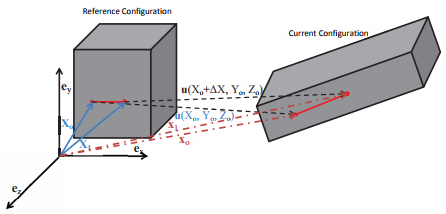Figure 3.3: Schematic of the deformation of a line oriented along ex of length ∆X.

where ux(X, Y, Z), uy(X, Y, Z) and uz(X, Y, Z) are some known functions of the X, Y and Z the coordinates of the material particles in the reference configuration.

3. It is assumed that the components of the displacement can be approximated as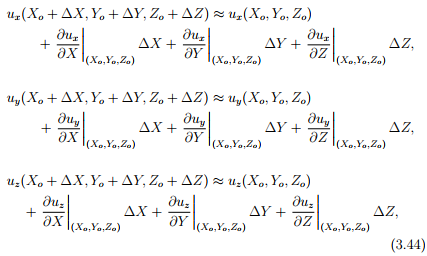where the displacement of the material particle occupying the point Xo is u(Xo, Yo, Zo) and that displacement of the material particle that is occupying, X1 is u(Xo + ∆X, Yo + ∆Y, Zo + ∆Z). Here it is pertinent to point out that the above is a truncated Taylor’s series. Since, we are interested in arbitrarily small values of ∆X, ∆Y , ∆Z, we have truncated the Taylor’s series after the first term. However, this is not a valid approximation for many functions. For example, functions of the form aX3/2 , where a is a constant defined over the domain, say 0.01 ≤ X ≤ 0.1 the value of the derivatives greater then second order would be more than the first order derivative. Hence, the expression derived based on this assumption, though intuitive has its limitations.
First, we are interested in finding how the length of the straight line of length ∆X oriented along the ex direction in the reference configuration of the body, as shown in the figure 3.3 has changed. With the above assumptions, the deformed length of the straight line of length ∆X oriented along the ex direction in the reference configuration is given by,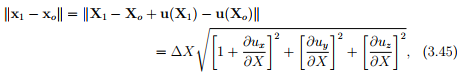where X1 = Xo + ∆Xex, xo denotes the current position vector of the material particle whose position in the reference configuration is Xo, x1 denotes the current position vector of the material particle whose position in the reference configuration is Xand use is made of equation (3.44). Now, if the magnitude of the components of the gradient of the displacement are small (say of the magnitude of 10−3 ), then equation (3.45) could be approximately calculated as,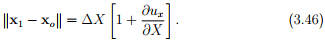Hence, the stretch ratio defined as the ratio of the current length to the undeformed length for a line element oriented along the ex direction is,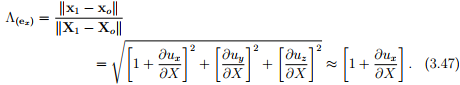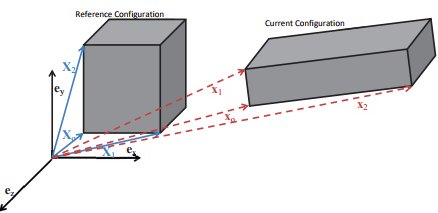Figure 3.4: Schematic of the deformation of a face of the cube.

which can be approximately computed as,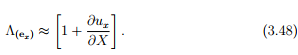when the components of the displacement gradient are small. Following a similar procedure, one can determine how the length of line elements oriented along any direction changes. We shall derive a general expression for the same later in section 3.6.1. Next we are interested in finding how the area of a face of a small cuboid changes due to deformation. Let us assume that the face of the cuboid whose normal coincides with the ez basis is of interest and the sides of this face of the cuboid are of length ∆X along the ex direction and ∆Y along the ey direction. Thus, as shown in figure 3.4, Xo (= Xoex + Yoey + Zoez), X1 (= (Xo+∆X)ex+Yoey+Zoez) and X2 (= Xoex+(Yo+∆Y )ey+Zoez) denote the position vector of the three corners of the face of the cuboid whose normal is ez in the reference configuration and xo, x1 and x2 denote the position of the same three corners of the face of the cuboid in the current configuration. For the same three assumptions listed above, the deformed area of this face of the cuboid is given by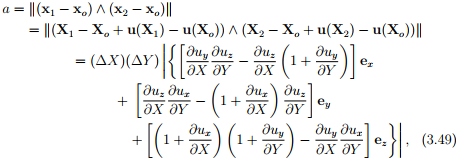and the orientation of the normal to this deformed face is computed as,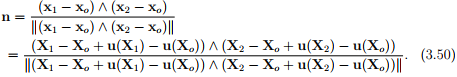Recollect that in chapter 2 we mentioned that the cross product of two vectors characterizes the area of the parallelogram spanned by them. We have made use of this to obtain the above expressions.
Finally, we find the volume of the deformed cuboid. the box product of three vectors yields the volume of the parallelepiped spanned by them, the deformed volume of the cuboid is given by,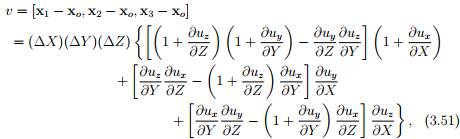where xo, x1, x2 and x3 are the position vectors of the material particles in the current configuration corresponding to whose position vector in the reference configuration are
Xo (= Xoex+Yoey +Zoez), X1 (= (Xo+∆X)ex+ Yoey + Zoez), X(= Xoex + (Yo + ∆Y )ey + Zoez) and X(= Xoex + Yoey + (Zo + ∆Z)ez) respectively.

When the magnitude of the components of the displacement gradient are small, then the deformed volume of the cuboid could be computed from equation (3.51) as,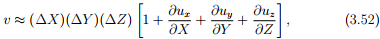by neglecting the quadratic and cubic terms in equation (3.51).

Transformation of curves
We know the position vector of any particle belonging to the body at various instances of time, t. But now we are interested in finding how a set of contiguous points forming a curve changes its shape. That is, we are interested in finding how a circle inscribed in the reference configuration changes its shape, say into an ellipse, in the current configuration. As we have required the deformation field to be one to one, closed curves like circle, ellipse, will remain as closed curves and open curves like straight line, parabola remains open. The position vectors of the particles that occupy a curve can be described using a single variable, say ξ. For example, a circle of radius R in the plane whose normal coincides with ez, as shown in figure 3.5a would be described as (R cos(ξ), R sin(ξ), Zo), where R and Zare constants and 0 ≤ ξ ≤ 2π.
Consider a material curve (or a curve in the reference configuration), X = Γ(ξ) ⊂ Br, where ξ denotes a parametrization. The material curve by virtue of it being defined in the reference configuration is not a function of time. During a certain motion, the material curve deforms into another curve called the spatial curve, x = γ(ξ, t) ⊂ Bt , (see figure 3.5b). The spatial curve at a fixed time t is then defined by the parametric equation

x = γ(ξ, t) = χ(Γ(ξ), t).                      (3.53)

We denote the tangent vector to the material curve as ∆X and the tangent vector to the spatial curve as ∆x and are defined by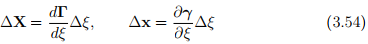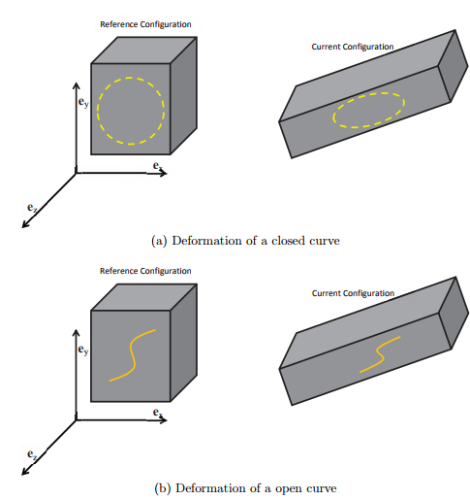Figure 3.5: Curves in the reference configuration deforming into another curve in the current configuration

By using (3.53) and the chain rule we find that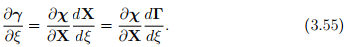Hence, from equation (3.54) and the definition of the deformation gradient, (3.11) we find that

x = F∆X.                                                                     (3.56)

Expression (3.56) clearly defines a linear transformation which generates a vector ∆x by the action of the second-order tensor F on the vector ∆X. In summary: material tangent vectors map into spatial tangent vectors via the deformation gradient. This is the physical significance of the deformation gradient.

In the literature, the tangent vectors ∆x and ∆X, in the current and reference configuration are often referred to as the spatial line element and the material line element, respectively. This is correct only when the curve in the reference and current configuration is a straight line.

Next, we introduce the concept of stretch ratio which is defined as the ratio between the current length to its original length. When we say original length, we mean undeformed length, that is the length of the fiber when it is not subjected to any force. It should be pointed out at the outset that many a times the undeformed length would not be available and in these cases it is approximated as the length in the reference configuration.

In general, the stretch ratio depends on the location and orientation of the material fiber for which it is computed. Thus, we can fix the orientation of the material fiber in the reference configuration or in the current configuration. Corresponding to the configuration in which the orientation of the fiber is fixed we have two stretch measures. Recognize that we can fix the orientation of the fibers in only one configuration because its orientation in others is determined by the motion.

First, we consider deformations in which any straight line segments in the reference configuration gets mapped on to a straight line segment in the current configuration. Such a deformation field which maps straight line segments in the reference configuration to straight line segments in the current configuration is called homogeneous deformation. We shall in section 3.10, illustrate that when the deformation is homogeneous, the Cartesian components of the deformation gradient would be a constant. As a consequence of assuming the curve to be a straight line, the tangent and secant to the curve is the same. Hence, saying that we are considering a material fiber3 of length ∆L initially oriented along A, (where A is a unit vector) and located at P, is same as saying that we are studying the influence of the deformation on the tangent vector, ∆LA at P. Due to some motion of the body, the material particle that occupied the point P will occupy the point p ∈ Bt and the tangent vector ∆LA is going to be mapped on to a tangent vector with length, say ∆l and orientation a. From (3.56) we have

∆la = ∆LFA.                                                                                     (3.57)

Dotting the above equation with ∆la, we get

(∆l)2 = (∆L)2FA � FA = (∆L)2FtFA � A,              (3.58)

where we have used the fact that a is a unit vector, the relation (3.57) and the definition of transpose. Defining the right Cauchy-Green deformation tensor, C as

C = FtF,                        (3.59)

and using equation (3.58) we obtain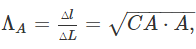(3.60)

where ΛA represents the stretch ratio of a line segment initially oriented along A. The stretch ratio, ΛA is the one that could be directly determined in an experiment. However, there is no reason why we should restrict our studies to line segments which are initially oriented along a given direction. We can also study about material fibers that are finally oriented along a given direction. Towards this, we invert equation (3.57) to get

∆LA = ∆lF−1 a.                                                                             (3.61)

Dotting the above equation with ∆LA, we get

(∆L)2 = (∆l)2F−1 a � F−1 a = (∆l)2F −tF−1 a � a,                             (3.62)

using arguments similar to that used to get (3.58) from (3.57). Defining the left Cauchy-Green deformation tensor or the Finger deformation tensor, B as

B = FFt ,                               (3.63)

 3A material fiber is also sometimes called as infinitesimal line elements.

and using equation (3.62) we obtain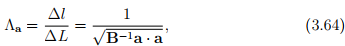where Λa represents the stretch ratio of a fiber finally oriented along a.
Next, we would like to compute the final angle between two two straight line segments initially oriented along A1 and A2. Recognizing that the angle between two straight line segments, α is computed using the expression: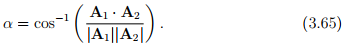Using (3.65) and similar arguments as that used to obtain the stretch ratio, it can be shown that the final angle, αf between the straight line segments initially oriented along Aand A2 is given by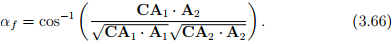Just, as in the case of stretch ratio, we can also study the initial angle, αi between straight line segments that are finally oriented along a1 and a2. For this case, following the same steps outlined above we compute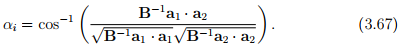We like to record that as in the case of stretch ratio, αi and αf depends on the orientation of the line segments. Next, let us see what happens when the deformation is inhomogeneous. For inhomogeneous deformation, the Cartesian components of the deformation gradient would vary spatially and the straight line segment in the reference configuration would have become a curve in the current configuration. Hence, distinction between the tangent and the secant has to be made. Now, the deformed length of the curve, ∆l corresponding to that of a straight line of length ∆L oriented along A is obtained from equation (3.56) as,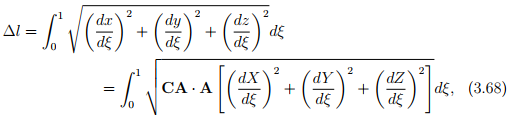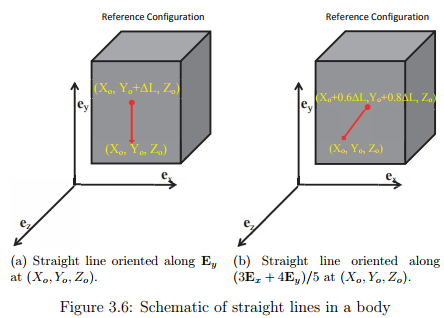where A is a constant vector,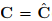(X(ξ), Y (ξ), Z(ξ)), (X(ξ), Y (ξ), Z(ξ)) denotes the coordinates of the material points that constitutes the straight line and ξ varies between 0 and 1 to parameterize the straight line. Thus, for a line oriented along say Ey direction of length ∆L, starting from a point (Xo, Yo, Zo), (see figure 3.6a) X(ξ) = Xo, Y (ξ) = Yo + ξ(∆L), Z(ξ) = Zo. For a line oriented along say (3Ex + 4EY )/5 of length ∆L, starting from (Xo, Yo, Zo), (see figure 3.6b) X(ξ) = Xo +3ξ(∆L)/5, Y (ξ) = Yo +4ξ(∆L)/5, Z(ξ) = Zo. In fact, if one relaxes the assumption that A is a constant in equation (3.68) then the straight line in the reference configuration could be a curve and the deformed length could still be calculated from (3.68).
Hence, the stretch ratio for the case of inhomogeneous deformation is,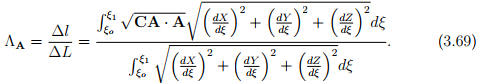Transformation of areas
Having seen how points, curves, tangent vectors in the reference configuration gets mapped to points, curves and tangent vectors in the current configuration, we are now in a position to look at how surfaces get mapped. Of interest, is how a unit vector, N normal to an infinitesimal material surface element ∆A map on to a unit vector n normal to the associated infinitesimal spatial surface element ∆a.

Let Sr denote the material surface in Br that is of interest. Then, an element of area ∆A at a point P on Sr is defined through the vectors ∆X and ∆Y, each tangent to Sr at P, as ∆AN = ∆X ∧ ∆Y where N is a unit vector normal to Sr at P. Due to some motion of the body, the material surface Sr gets mapped on to another material surface St in Bt , the material particle that occupied the point P on Sr gets mapped onto a point p on St and the tangent vectors ∆X and ∆Y gets mapped on to another pair of tangent vectors at p denoted by ∆x and ∆y. Thus, we have

∆AN = ∆X ∧ ∆Y,                 ∆an = ∆x ∧ ∆y.                                                     (3.70)

Since, ∆X and ∆Y are tangent vectors we have ∆x = F∆X and ∆y = F∆Y. Therefore,

∆an = (F∆X) ∧ (F∆Y) = F*(∆X ∧ ∆Y) = det(F)F−tN∆A,                                   (3.71)

where we have made use of (2.88) and (2.90). Thus, the relation

∆an = det(F)F−tN∆A,                                                                                       (3.72)

called Nanson’s formula is an often used expression in continuum mechanics. Next, we are interested in computing the deformed area, a. For this case, from Nanson’s formula we obtain,

(∆a)n � (∆a)n = det(F)F−tN(∆A) � det(F)F−tN(∆A),                                            (3.73)

which simplifies to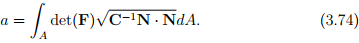on using the equation (3.59) and the fact that n is a unit vector. Notice that, once again the deformed area is determined by the right Cauchy-Green deformation tensor.

Transformation of volumes
Next, we seek to obtain the relation between elemental volumes in the reference and current configuration. An element of volume ∆V at a interior point P in reference configuration is given by the scalar triple product [∆X, ∆Y, ∆Z] where ∆X, ∆Y and ∆Z are vectors oriented along the sides of an infinitesimal parallelepiped. Since, the point under consideration is interior, we can always find three material curves such that they pass through the point P and ∆X, ∆Y and ∆Z are its tangent vectors at P. Now say due to some motion of the body, the material particle that occupied the point P, now occupies the point p, then the curves under investigation would pass through p and the tangent vectors of these curves at this point p would now be ∆x, ∆y, ∆z. Hence,

∆v = [∆x, ∆y, ∆z] = [F∆X, F∆Y, F∆Z] = det(F)[∆X, ∆Y, ∆Z] = det(F)∆V,              (3.75)

where we have used the definition of the determinant (2.77). Since, the coordinate basis vectors for the reference and current configuration have the same handedness, det(F) ≥ 0. Further since, we require the mapping of the body from one configuration to the other be one to one, material particles in a parallelepiped cannot be mapped on to a surface. Hence, det(F) ≠ 0. Therefore det(F) > 0. Thus, for a vector field to be a deformation field, det(F) > 0.

Offer running on EduRev: Apply code STAYHOME200 to get INR 200 off on our premium plan EduRev Infinity!

## Advanced Solid Mechanics - Notes, Videos, MCQs & PPTs

42 videos|61 docs

,

,

,

,

,

,

,

,

,

,

,

,

,

,

,

,

,

,

,

,

,

,

,

,

;